Next: Fine Structure of Hydrogen Up: Time-Independent Perturbation Theory Previous: Degenerate Perturbation Theory

# Linear Stark Effect

Returning to the Stark effect, let us examine the effect of an external electric field on the energy levels of thestates of a hydrogen atom. There are four such states: an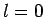state, usually referred to as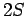, and three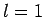states (with), usually referred to as 2P. All of these states possess the same unperturbed energy,. As before, the perturbing Hamiltonian is(956)

According to the previously determined selection rules (i.e.,, and), this Hamiltonian couples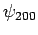and. Hence, non-degenerate perturbation theory breaks down when applied to these two states. On the other hand, non-degenerate perturbation theory works fine for theand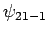states, since these are not coupled to any otherstates by the perturbing Hamiltonian.

In order to apply perturbation theory to theandstates, we have to solve the matrix eigenvalue equation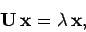(957)

where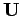is the matrix of the matrix elements of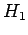between these states. Thus,(958)

where the rows and columns correspond toand, respectively. Here, we have again made use of the selection rules, which tell us that the matrix element ofbetween two hydrogen atom states is zero unless the states possess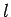quantum numbers which differ by unity. It is easily demonstrated, from the exact forms of the 2S and 2P wavefunctions, that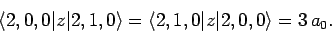(959)

It can be seen, by inspection, that the eigenvalues ofare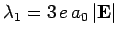and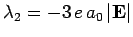. The corresponding normalized eigenvectors are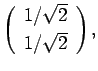(960)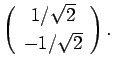(961)

It follows that the simultaneous eigenstates ofandtake the form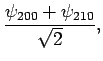(962)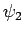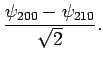(963)

In the absence of an external electric field, both of these states possess the same energy,. The first-order energy shifts induced by an external electric field are given by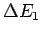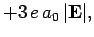(964)(965)

Thus, in the presence of an electric field, the energies of states 1 and 2 are shifted upwards and downwards, respectively, by an amount. These states are orthogonal linear combinations of the originalandstates. Note that the energy shifts are linear in the electric field-strength, so this effect--which is known as the linear Stark effect--is much larger than the quadratic effect described in Sect. 12.5. Note, also, that the energies of theandstates are not affected by the electric field to first-order. Of course, to second-order the energies of these states are shifted by an amount which depends on the square of the electric field-strength (see Sect. 12.5).Next: Fine Structure of Hydrogen Up: Time-Independent Perturbation Theory Previous: Degenerate Perturbation Theory
Richard Fitzpatrick 2010-07-20